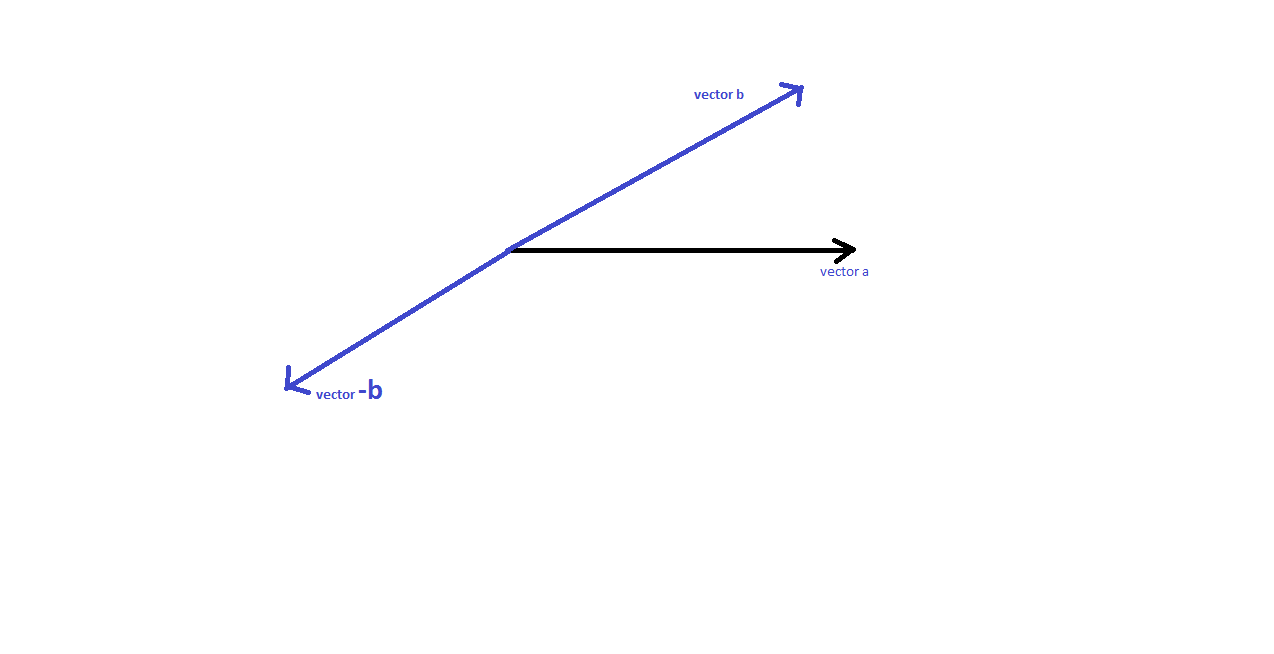# In the formula for the mag of the resultant of the difference of 2 vectors sq root(a^2 + b^2 - 2abcos\$)is \$ the angle between a and b or between a and -b?

10 years ago

it is the angle between a and -b.

as we know,we know that a+b=sq.root(a2+b2+2abcosA)

where A is the angle between a and b.

here he asked the difference so we shoud replace b with -b.

then,a-b=sq.root(a2+b2+2a(-b)cosA)

threfore here A=angle between a and-b.

10 years ago

Hi Shayan,

Analyse the problem and try to simplify it,

The question says mag of resultant of 2 Vectors = |a - b|

Now |a-b|2 = (a-b).(a-b) = |a|2+|b|2 - 2a.b

or |a-b| = (|a|2+|b|2 - 2a.b)1/2.

So \$ is certainly the angle between vector "a" and vector "b".

Regards,

10 years ago

Shayan,

If in the expression you had

(a^2 + b^2 + 2(a)(-b)cos\$), then it would have been the angle between a and -b.

These kinds of traps are generally set by IITs in the exam, and one needs to be carefule.

In this case it is the angle between a and b only.

Regards,

10 years ago

Hi Shayan,

No worries.

The question requires one to find if "\$" is the angle between "a" & "b" or "a" & "-b"

So you have to compare the final result with the given expression in the question.

You have |a-b| = sqrt{ a^2 + b^2 - 2a.b} as in the above working.

Compare this with the expression in your question.

we will have a.b = ab(cos\$)

which shows that \$ is the abgle between "a" vector and "b" vector.

Hope this helps.

Best Regards,